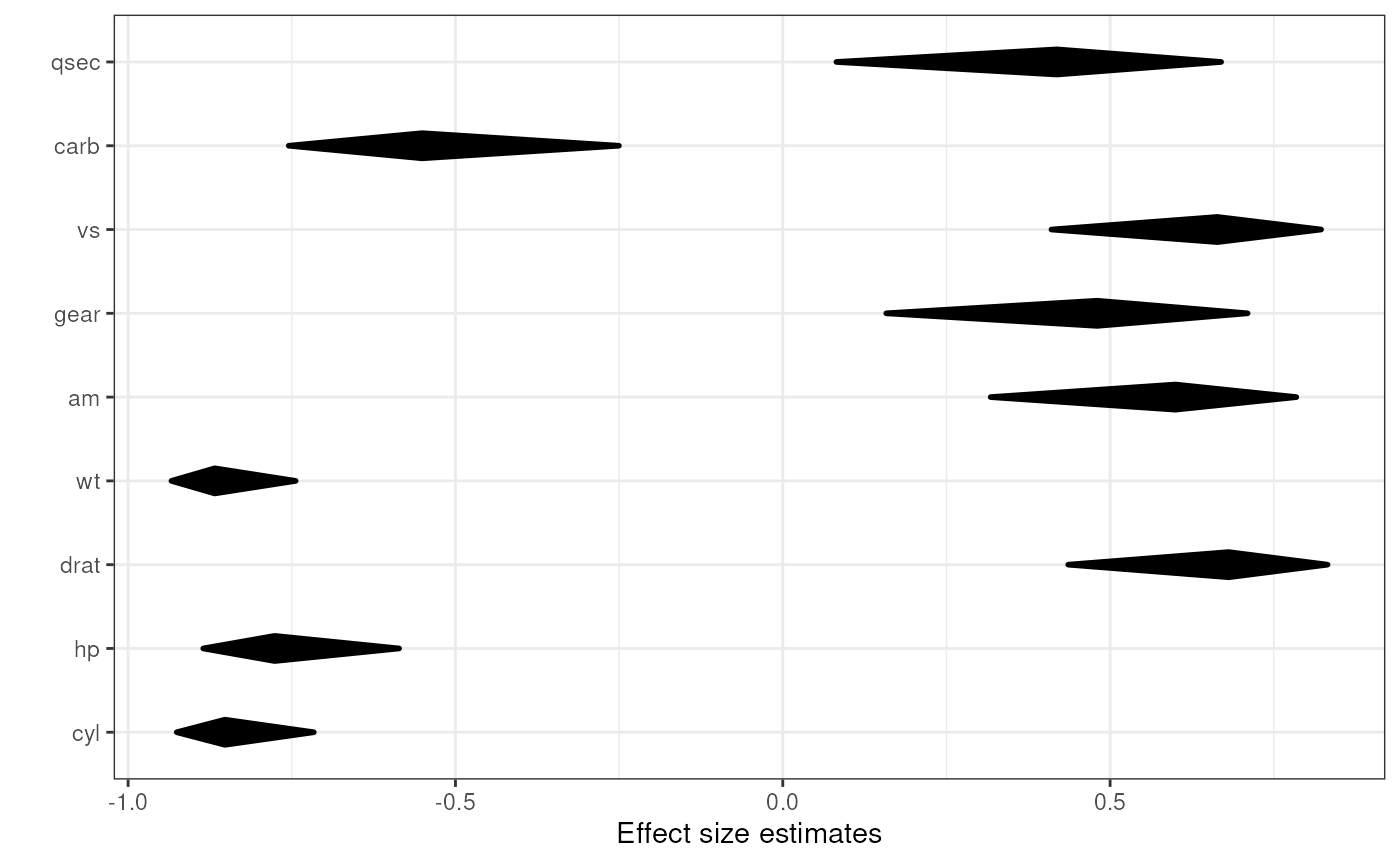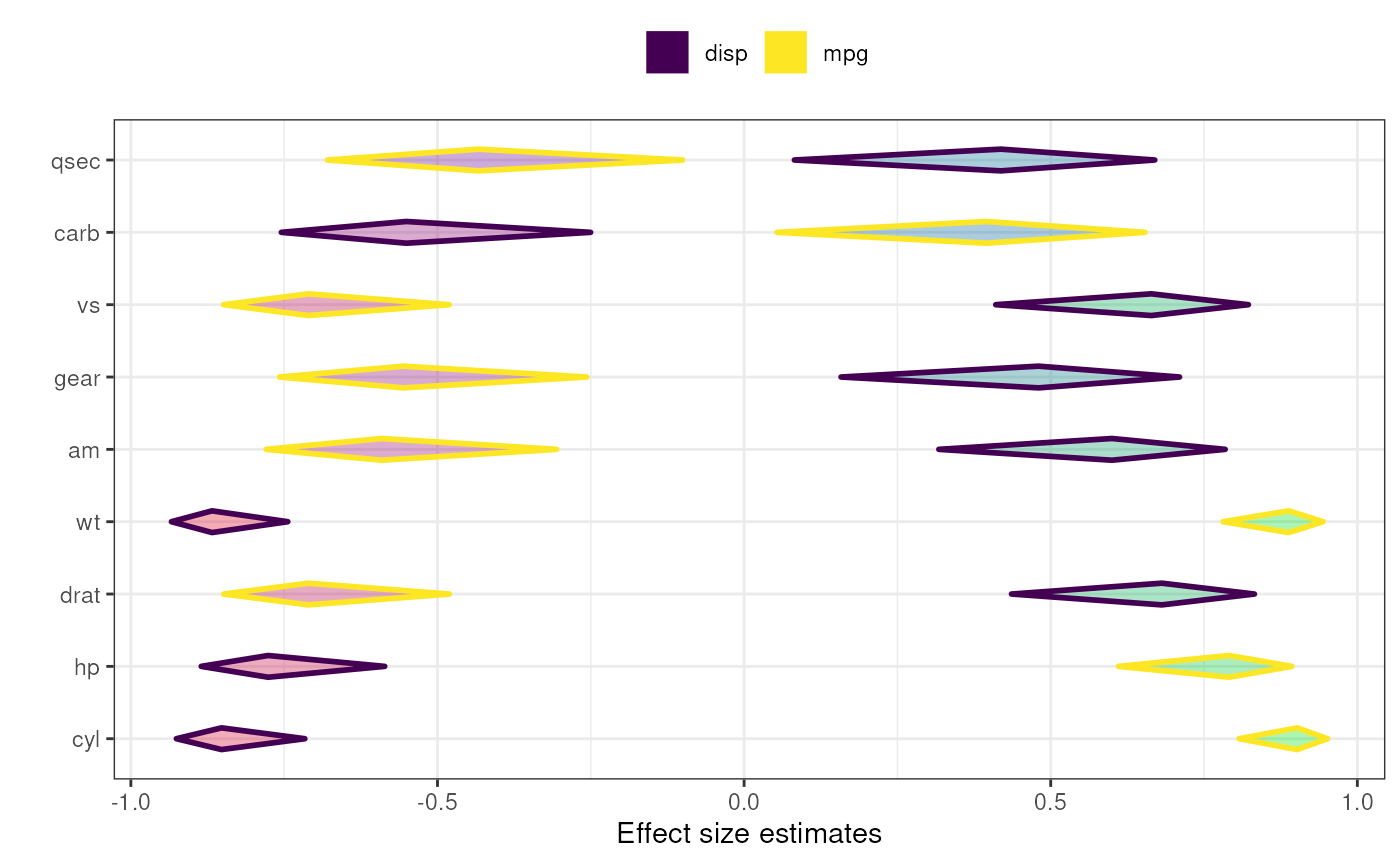This function produces is a diamondplot that plots the confidence intervals for associations between a number of covariates and a criterion. It currently only supports the Pearson's r effect size metric; other effect sizes are converted to Pearson's r.

associationsDiamondPlot(dat, covariates, criteria, labels = NULL,
criteriaLabels = NULL, decreasing = NULL, sortBy = NULL,
conf.level = 0.95,
criteriaColors = viridis::viridis(length(criteria)),
criterionColor = "black", returnLayerOnly = FALSE, esMetric = "r",
multiAlpha = 0.33, singleAlpha = 1, showLegend = TRUE,
xlab = "Effect size estimates", ylab = "",
theme = ggplot2::theme_bw(), lineSize = 1, outputFile = NULL,
outputWidth = 10, outputHeight = 10, ggsaveParams = list(units =
"cm", dpi = 300, type = "cairo"), ...)

associationsToDiamondPlotDf(dat, covariates, criterion, labels = NULL,
decreasing = NULL, conf.level = 0.95, esMetric = "r")

## Arguments

dat The dataframe containing the relevant variables. The covariates: the list of variables to associate to the criterion or criteria, usually the predictors. The criteria, usually the dependent variables; one criterion (one dependent variable) can also be specified of course. The helper function associationsToDiamondPlotDf always accepts only one criterion. The labels for the covariates, for example the questions that were used (as a character vector). The labels for the criteria (in the legend). Whether to sort the covariates by the point estimate of the effect size of their association with the criterion. Use NULL to not sort at all, TRUE to sort in descending order, and FALSE to sort in ascending order. When specifying multiple criteria, this can be used to indicate by which criterion the items should be sorted (if they should be sorted). The confidence of the confidence intervals. The colors to use for the different associations can be specified in criteriaColors. This should be a vector of valid colors with at least as many elements as criteria are specified in criteria. If only one criterion is specified, the color in criterionColor is used. Whether to return the entire object that is generated, or just the resulting ggplot2 layer. The effect size metric to plot - currently, only 'r' is supported, and other values will return an error. The transparency (alpha channel) value of the diamonds for each association can be specified in multiAlpha, and if only one criterion is specified, the alpha level of the diamonds can be specified in singleAlpha. Whether to show the legend. The label to use for the x and y axes (for duoComparisonDiamondPlot, must be vectors of two elements). Use NULL to not use a label. The ggplot() theme to use. The thickness of the lines (the diamonds' strokes). A file to which to save the plot. Width and height of saved plot (specified in centimeters by default, see ggsaveParams). Parameters to pass to ggsave when saving the plot. Any additional arguments are passed to diamondPlot() and eventually to ggDiamondLayer().

A plot.

## Details

associationsToDiamondPlotDf is a helper function that produces the required dataframe.

This function can be used to quickly plot multiple confidence intervals.

diamondPlot(), ggDiamondLayer(), behaviorchange::CIBER()

## Examples


### Simple diamond plot with correlations
### and their confidence intervals

associationsDiamondPlot(mtcars,
covariates=c('cyl', 'hp', 'drat', 'wt',
'am', 'gear', 'vs', 'carb', 'qsec'),
criteria='mpg');### Same diamond plot, but now with two criteria,
### and colouring the diamonds based on the
### correlation point estimates: a gradient
### is created where red is used for -1,
### green for 1 and blue for 0.

associationsDiamondPlot(mtcars,
covariates=c('cyl', 'hp', 'drat', 'wt',
'am', 'gear', 'vs', 'carb', 'qsec'),
criteria=c('mpg', 'disp'),
generateColors=c("red", "blue", "green"),
fullColorRange=c(-1, 1));